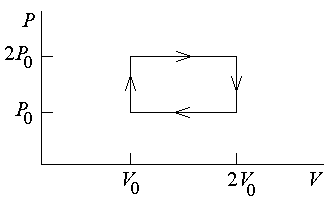First Name: ___________________ Last Name: ____________________ Section: _________

April 24, 1998 Physics 103

Exam 3

Print your name and section clearly on all five pages. (If you do not know your section number, write your TAs name.) Show all work in the space immediately below each problem. Your final answer must be placed in the box provided. Problems will be graded on reasoning and intermediate steps as well as on the final answer. Be sure to include units wherever necessary, and the direction of vectors. Each problem is worth 25 points. In doing the problems, try to be neat. Check your answers to see that they have the correct dimensions (units) and are the right order of magnitudes. You are allowed one 8½ x 11" sheet of notes and no other references. The exam lasts exactly 50 minutes.

(Do not write below)

SCORE:

Problem 1: __________

Problem 2: __________

Problem 3: __________

Problem 4: __________

TOTAL: ___________

First Name: ___________________ Last Name: ____________________ Section: _________

1. A horizontal garden hose has an inside diameter of 2 cm and a nozzle with a diameter of 0.5 cm, through which 5 liters of water flows per minute.

a. Calculate the speed of the water leaving the nozzle. (9 pts.)

b. Calculate the speed of the water in the large part of the hose. (7 pts.)

c. By how much does the water pressure in the pipe exceed the pressure of the atmosphere? (9 pts.)

First Name: ___________________ Last Name: ____________________ Section: _________

2. A weather balloon with a diameter of 1 m is filled with helium gas at a pressure of 1 atmosphere and 20°C. Helium has a molecular weight of 4 g/mole, and air has an average molecular weight of 29 g/mole.

a. How many molecules of helium are in the balloon? (8 pts.)

b. What is the buoyant force on the balloon at sea level? (9 pts.)

c. What is the root mean square speed of the helium molecules? (8 pts.)

First Name: ___________________ Last Name: ____________________ Section: _________

3. Fifty grams of ice at 0° C is added to 200 ml of water at 25° C in a thermally insulated container open to the atmosphere.

1. What is the temperature of the mixture after it has reached thermal equilibrium? (9 pts.)

b. How much heat must be added to the resulting mixture to completely boil it away at sea level? (8 pts.)

c. What is the minimum cost of boiling the mixture on an electric stove if electricity costs 7 cents per kilowatt-hour? (8 pts.)

First Name: ___________________ Last Name: ____________________ Section: _________

4. A heat engine that contains 1 mole of an ideal gas initially at pressure P0, volume V0, and temperature T0 = 0° C cycles through pressure and volume as shown below:a. How many joules of work are done in each cycle of the engine? (10 pts.)

b. What maximum temperature does the gas reach during the cycle? (10 pts.)

c. What is the change in internal energy of the gas after one complete cycle? (5 pts.)